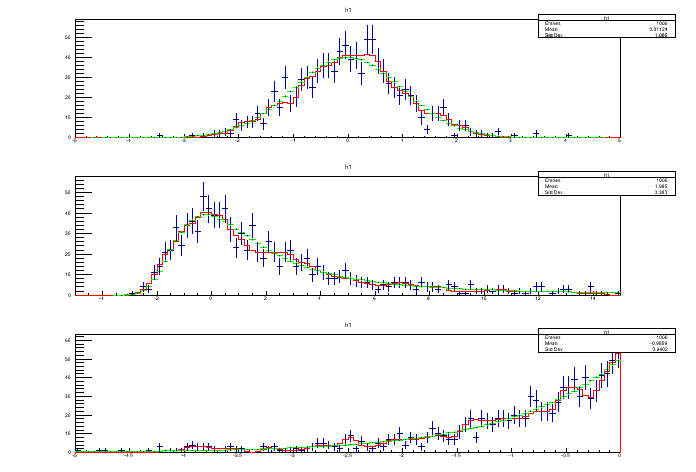# Test Smooth¶

Histogram smoothing.

Author: Rene Brun
This notebook tutorial was automatically generated with ROOTBOOK-izer from the macro found in the ROOT repository on Thursday, May 19, 2022 at 08:16 AM.

In :
int ipad = 1;
TCanvas * c1 = 0;


A helper function is created:

In :
%%cpp -d
void smooth_hist(const char * fname, double xmin, double xmax, int n1, int n2) {

std::cout << "smoothing a " << fname << " histogram" << std::endl;

TH1D * h1 = new TH1D("h1","h1",100,xmin,xmax);
TH1D * h2 = new TH1D("h2","h2",100,xmin,xmax);
h1->FillRandom(fname,n1);

TH1D * h1_s = new TH1D(*h1);
h1_s->SetName("h1_s");
h1_s->Smooth();

h2->FillRandom(fname,n2);

double p1 = h1->Chi2Test(h2,"");
double p2 = h1_s->Chi2Test(h2,"UU");
if (p2 < p1) Error("testSmooth","TH1::Smooth is not working correctly - a worst chi2 is obtained");

std::cout << " chi2 test non-smoothed histo " << p1 <<  std::endl;
std::cout << " chi2 test smoothed histo     " << p2 <<  std::endl;

double a1 = h1->AndersonDarlingTest(h2);
double a2 = h1_s->AndersonDarlingTest(h2);

std::cout << " AD test non-smoothed histo " << a1 <<  std::endl;
std::cout << " AD test smoothed histo     " << a2 <<  std::endl;

double k1 = h1->KolmogorovTest(h2);
double k2 = h1_s->KolmogorovTest(h2);

std::cout << " KS test non-smoothed histo " << k1 <<  std::endl;
std::cout << " KS test smoothed histo     " << k2 <<  std::endl;

h1->Draw("E");
h1_s->SetLineColor(kRed);
h1_s->Draw("same");
h2->Scale(double(n1)/n2);
h2->SetLineColor(kGreen);
h2->Draw("same");
}


Arguments are defined.

In :
int n1 = 1000;
int n2 = 1000000;

In :
TH1::AddDirectory(false);

c1  = new TCanvas();
c1->Divide(1,3);

smooth_hist("gaus",-5,5,n1,n2);
smooth_hist("landau",-5,15,n1,n2);
smooth_hist("expo",-5,0,n1,n2);

smoothing a gaus histogram
chi2 test non-smoothed histo 5.15629e-11
chi2 test smoothed histo     1
KS test non-smoothed histo 0.933145
KS test smoothed histo     0.770148
smoothing a landau histogram
chi2 test non-smoothed histo 0.966464
chi2 test smoothed histo     1
KS test non-smoothed histo 0.7146
KS test smoothed histo     0.764859
smoothing a expo histogram
chi2 test non-smoothed histo 0.364215
chi2 test smoothed histo     0.999855
KS test non-smoothed histo 0.966402
KS test smoothed histo     0.799221

Info in <TH1D::Chi2TestX>: There is a bin in h1 with less than 1 event.

Info in <TH1D::Chi2TestX>: There is a bin in h1 with less than 1 event.

Info in <TH1D::Chi2TestX>: There is a bin in h1 with less than 1 event.

Info in <TH1D::Chi2TestX>: There is a bin in h1 with less than 1 event.

Info in <TH1D::Chi2TestX>: There is a bin in h1 with less than 1 event.

Info in <TH1D::Chi2TestX>: There is a bin in h1 with less than 1 event.



Draw all canvases

In :
gROOT->GetListOfCanvases()->Draw()